# AI For Trading: SMB 和 HML (55)

## SMB

To create a theoretical portfolio representing size, we could go long the bottom 10th percentile of stocks by market cap (long small cap stocks) and go short stocks above the 90th percentile (go short the large cap stocks). We could assume an equal dollar amount invested in each stock. In the above example, we are dividing by 2 to take the average return of going long small cap stocks and going short large cap stocks.

It's also common to compute the spread between two portfolios. One portfolio contains the small cap stocks, and the other portfolio contains the large cap stocks. In this case, we'd just take the difference between the returns of the two portfolios.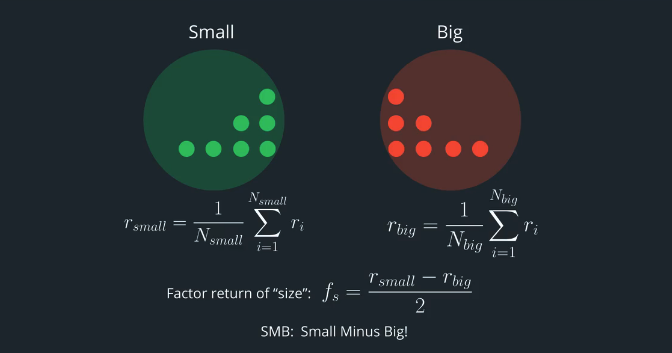SMB = Small minus Big

## HML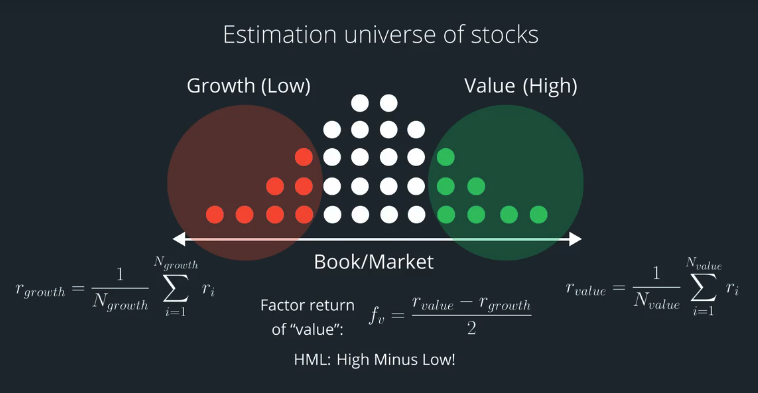HML = High minus Low

## Fama French SMB and HML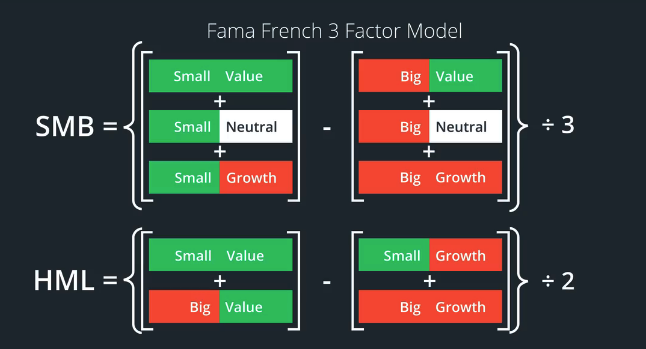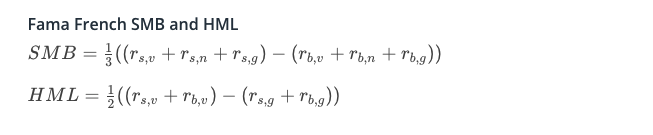## Fama French Risk Model

### Matrix of Factor Returns

Calculate the covariance matrix using the time series of factor returns.

### Matrix of Factor Exposures

Use a multiple regression to estimate the factor exposures.

### Specific Variance

Calculate the actual minus estimated returns as the specific return. The variance of that time series is an estimate of specific variance.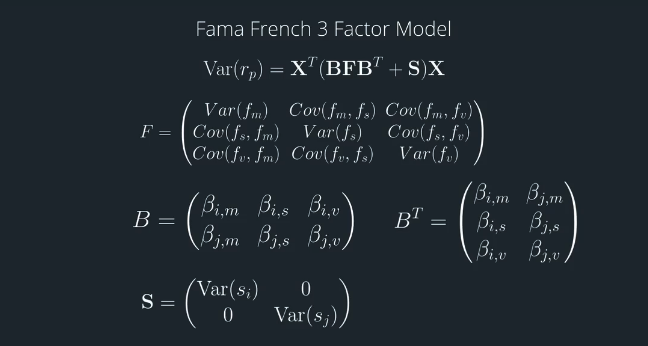## Categorical Factors

When handling categorical variables, we can make each unique value within a category be its own variable. In this example, the country variable becomes "country_usa", "country_india", "country_brazil" etc. Then assign a value to each of these variables to represent how "exposed" the company is to each country.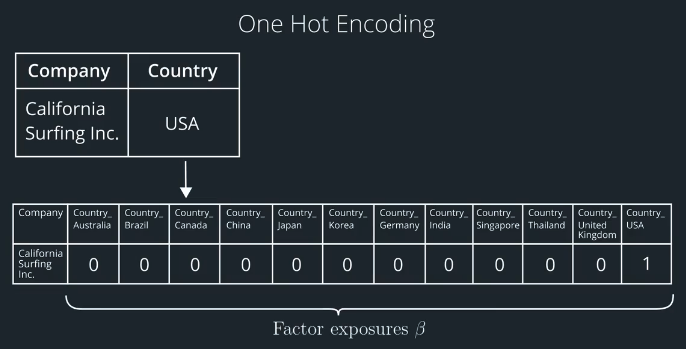### Estimating Factor Return

If we collect a cross-section of multiple stocks for a single time period, then we'll have pairs of stock returns and factor exposures. We can use regression to estimate the factor return for that single time period. Then repeat over multiple time periods to get a time series of factor returns.